Do you like the article?
Share it with others —
Use new possibilities of MetaTrader 5

#### Similar articles# Genetic Algorithms: Mathematics

MetaTrader 4Tester | 16 June 2006, 11:1814 7532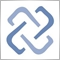Genetic algorithms are used for optimization purposes. An example of such purpose can be neuronet learning, i.e., selection of such weight values that allow reaching the minimum error. At this, the genetic algorithm is based on the random search method. The principal trouble with random search is the fact that we cannot be aware of how much time it takes to solve the problem. To avoid significant wastes of time,  they apply methods developed in biology, namely, the methods prepared in studies of origin of species and evolution. Only the fittest animals are known to survive during evolution. As a result, the fitness of the population grows what enables it to adjust the dynamic environment.

The algorithm of the kind was first proposed by John H. Holland, University of Michigan, USA, in 1975. It was named the Holland's Reproductive Plan, and this underlay almost all types of genetic algorithms. However, before we take a look more closely at this plan, we will discuss the matter how realities can be encoded to be used in genetic algorithms.

## Object Presentation

We know from biology that any organism can be represented as its phenotype, which determines, in fact, what this object is in the real world, and its genotype, which contains the entire information about this object at its set of chromosomes. At this, every gene, i.e. every element of the genotype information, reflects in the phenotype. Thus, to solve our problems, we have to present every character of the object in such a form, which can be used in a genetic algorithm. The mechanisms of the genetic algorithm will further function at the genotype level, with no need of information about the object's inner pattern, what provides  the wide use of these algorithms for multitude of very different tasks.

The bit strings are used for presentation of the object's genotype in the most widely met variation of genetic algorithm. At this, one gene of the object's genotype corresponds with every attribute of the object in its phenotype. Gene is a fixed-length bit string that represents the value of this characteristic.

## Encoding of Integer Attributes

The simplest way of encoding such attributes is to use its bit value. Then it will be rather simple to use a gene of a certain length sufficient to represent all possible values of such an attribute. But, unfortunately,  this way of encoding has its disadvantages. The key disadvantage is that the neighboring numbers differ from each other in values of several bits. Thus, 7 and 8 in their bit representation differ in 4 positions what makes functioning of the genetic algorithm difficult and increases the time it takes for its convergence. To avoid this, it is better to use coding where the neighboring numbers differ from each other by fewer positions, ideally - by the one-bit value. Such coding is represented by the Gray code that is advisable to be used in the realization of a genetic algorithm. The Gray code values are given in the table below:

Binary Coding Gray Coding
Decimal Code Binary Code
0 0000 0h 0 0000 0h
1 0001 1h 1 0001 1h
2 0010 2h 3 0011 3h
3 0011 3h 2 0010 2h
4 0100 4h 6 0110 6h
5 0101 5h 7 0111 7h
6 0110 6h 5 0101 5h
7 0111 7h 4 0100 4h
8 1000 8h 12 1100 Ch
9 1001 9h 13 1101 Dh
10 1010 Ah 15 1111 Fh
11 1011 Bh 14 1110 Eh
12 1100 Ch 10 1010 Ah
13 1101 Dh 11 1011 Bh
14 1110 Eh 9 1001 9h
15 1111 Fh 8 1000 8h
Table 1. Concordance of Binary Codes and Gray Codes.

Thus, when encoding an integer attribute, we divide it into tetrads and transform each tetrad according to the Gray coding rules.

In practical realizations of genetic algorithms, there is usually no need to transform the attribute values into the gene values. In practice, the inverse problem takes place where one has to find the value of the attribute by the value of the corresponding gene.

Thus, the task of decoding the values of genes, which have integer attributes, is trivial.

## Encoding of Floating-Point Attributes

The simplest way of encoding here seems to be the use of bit representation. This way has the same disadvantages as that for integers, though. This is why, in practice, the following sequence of operations  will apply:

1. The entire interval of allowed values of the attribute is split into parts with the desired precision.
2. The gene value is taken as an integer that numbers the interval (using the Gray code).
3. The number that is the middle of this interval is taken as the parameter value.

Let us take a second look at the above sequence of operations in the following example:

Let us assume that the attribute values lie in the range of [0,1]. The range was split into 256 intervals for encoding. To encode their number, we will need 8 bit.  The gene value is, for example, 00100101bG (the capital letter G means that it is the Gray code). First, using the Gray code, let us find the corresponding interval number: 25hG->36h->54d. Now, let's check what interval corresponds with it. By simple calculations, we obtain the interval of [0,20703125, 0,2109375]. I.e., the value of parameter will be (0,20703125+0,2109375)/2=0,208984375.

## Encoding of Non-Numeric Data

The non-numeric data must be transformed into numbers before they are encoded. This is described in more details in the articles on our website, that describe the use of neural networks.

## How to Determine the Object's Phenotype by Its Genotype

To determine the object's phenotype (i.e., the values of the object's attributes), we just need to know the values of genes that correspond with these attributes (i.e., the object's genotype). At this, the integrity of genes that describe the object's genotype represents a chromosome. In some realizations, it is also named specimen. Thus, in the genetic algorithm realization, the chromosome represents a fixed-length bit string. At this, every interval of the string corresponds with a gene. The length of genes within a chromosome can be the same or different. The genes of the same length are used more frequently. Let us consider an example of a chromosome and interpretations of its value. Let the object have 5 attributes, every being encoded in a gene of 4-element length. Then the chromosome length is 5*4=20 bit:

 0010 1010 1001 0100 1101

We can determine the values of attributes now

Attribute Gene Value Binary Value of the Attribute Decimal Value of the Attribute
Attribute 1 0010 0011 3
Attribute 2 1010 1100 12
Attribute 3 1001 1110 14
Attribute 4 0100 0111 7
Attribute 5 1101 1001 9

## The Basic Genetic Operators

As is well known, the way in which the parental characters are bred true in the offsprings is very important in the evolution theory. In genetic algorithms, the crossover is a genetic operator used to vary the programming of a chromosome, or chromosomes, from one generation to the next. This operator works in the following way:

1. two units are selected in a population to be parents;
2. the break point is determined (randomly, as a rule);
3. the offspring is determined as concatenation of parts of the first and the second parent.

Let us have a look at functioning of this operator:

 Chromosome_1: 0000000000 Chromosome_2: 1111111111

Assume that the break point takes place after the 3rd bit of the chromosome, then:

 Chromosome_1: 0000000000 >> 000 1111111 Resulting_chromosome_1 Chromosome_2: 1111111111 >> 111 0000000 Resulting_chromosome_2

Then one of the resulting chromosomes is determined with a probability of 0.5 as an offspring.

The other genetic operator is to maintain the diversity in a population. It is called mutation operator. This operator that alters one ore more gene values in a chromosome from its initial state. Accordingly, every bit in a chromosome is inverted with a certain probability.

Besides, one more operator, named inversion, is used. It divides the chromosome into two parts, which then change places. This can be schematically represented as follows:

 000 1111111 >> 1111111 000

Theoretically, these two genetic operators are enough to make the genetic algorithm function. However, in practice, some additional operators are used, as well as modifications of these two operators. For example, there can be not only a single-point crossover (described above), but also a multipoint one. In the latter case, several break points (usually two) are created. Besides, the mutation operator performs the inversion of only one randomly selected bit of a chromosome in some implementations of the algorithm.

## Genetic Algorithm Flowchart

Now, with the knowledge of how to interpret the gene values, we can discuss how the genetic algorithm functions. Let us have a closer look at the genetic algorithm flowchart in its classical representation.

1. Initialize the start time, t=0. Form randomly the initial population that consists of k units. B0 = {A1,A2,…,Ak)
2. Calculate the fitness of each unit, FAi = fit(Ai) , i=1…k, and the fitness of the entire population, Ft = fit(Bt). The value of this function determines to what extent the unit described by this chromosome suits to solve the problem.
3. Select the Ac unit in the population. Ac = Get(Bt)
4. Select the second unit in the population with a certain probability (the crossover Pc probability), Аc1 = Get(Bt), and perform the crossover operator, Ac = Crossing(Ac,Ac1).
5. Perform the mutation operator with a certain probability (the mutation Pm probability), Ac = mutation(Ac).
6. Perform the inversion operator with a certain probability (the inversion Pi probability), Ac = inversion(Ac).
7. Place the obtained new chromosome into the new population, insert(Bt+1,Ac).
8. Steps 3 to 7 should be repeated k times.
9. Increase the current epoch number, t=t+1.
10. If the stop condition is met, terminate the loop. Otherwise, go to step 2.

Some stages of the algorithm need closer consideration.

Steps 3 and 4, the stage of parent chromosomes selection, play the most important role in successful functioning of the algorithm. There can be various possible alternatives at this. The most frequently used selection method is called roulette. When this method is used, the probability that this or that chromosome will be selected is determined by its fitness, i.e., PGet(Ai) ~ Fit(Ai)/Fit(Bt). The use of this method results in the increasing of the probability that attributes belonging to the most adjusted units will be propagated in the offsprings. Antoher frequently used method is the tournament selection. It consists in that several units (2, as a rule) are randomly selected among the population. The fittest unit will be selected as a winner.Besides, in some implementations of the algorithm, the so-called elitism strategy is used, which means that the best-adjusted units are guaranteed to enter the new population. This approach usually allows to accelerate the genetic algorithm convergence. The disadvantage of this strategy is the increased probability of the algorithm getting in the local minimum.

The determination of the algorithm stop criteria is another important point. Either the limitation of the algorithm functioning epochs or determination of the convergence of the algorithm (normally, through comparison of the population fitness in several epochs to the stop when this parameter is stabilized) are used as such criteria.

Translated from Russian by MetaQuotes Ltd.
Original article: https://www.mql5.com/ru/articles/1408

#### Other articles by this author

Last comments | Go to discussion (2)[Deleted] | 16 Nov 2006 at 22:04
I was wondering....
any code ready for MultiExpertSystems ?| 29 Dec 2006 at 12:33
redsnow:
I was wondering....
any code ready for MultiExpertSystems ?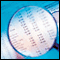One-Minute Data Modelling Quality Rating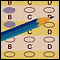How to Evaluate the Expert Testing Results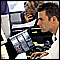Free-of-Holes Charts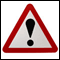Requirements Applicable to Articles Offered for Publishing at MQL4.com